Applied and Computational Mathematics
Volume 5, Issue 3, June 2016, Pages: 138-141

The Wiener Index and the Hosoya Polynomial of the Jahangir Graphs

Shaohui Wang1, 2, Mohammad Reza Farahani3, *, M. R. Rajesh Kanna4, Muhammad Kamran Jamil5, R. Pradeep Kumar6

1Department of Mathematics, University of Mississippi, University, MS, USA

2Department of Mathematics and Computer Science, Adelphi University, Garden City, NY, USA

3Department of Applied Mathematics, Iran University of Science and Technology (IUST) Narmak, Tehran, Iran

4Department of Mathematics, Maharani's Science College for Women, Mysore, India

5Department of Mathematics, Riphah Institute of Computing and Applied Sciences (RICAS), Riphah International University, Lahore, Pakistan

6Department of Mathematics, the National Institute of Engineering, Mysuru, India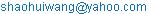(S. Wang)(M. R. Farahani)(M. R. R. Kanna)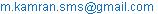(M. K. Jamil)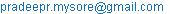(P. P. Kumar)

*Corresponding author

Shaohui Wang, Mohammad Reza Farahani, M. R. Rajesh Kanna, Muhammad Kamran Jamil, R. Pradeep Kumar. The Wiener Index and the Hosoya Polynomial of the Jahangir Graphs. Applied and Computational Mathematics. Vol. 5, No. 3, 2016, pp. 138-141. doi: 10.11648/j.acm.20160503.17

Received: April 21, 2016; Accepted: May 3, 2016; Published: July 13, 2016

Abstract: Let G be a simple connected graph having vertex set V and edge set E. The vertex-set and edge-set of G denoted by V(G) and E(G), respectively. The length of the smallest path between vertices u,v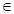V(G) is called the distance, d(u,v), between the vertices u,v. Mathematical chemistry is the area of research engaged in new application of mathematics in chemistry. In mathematics chemistry, we have many topological indices for any molecular graph, that they are invariant on the graph automorphism. In this research paper, we computing the Wiener index and the Hosoya polynomial of the Jahangir graphs J5,m for all integer number m≥3. The Wiener index is the sum of distances between all pairs of vertices of G as W(G)=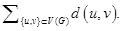And the Hosoya polynomial of G is H(G,x)=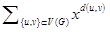, where d(u,v) denotes the distance between vertices u and v.

Keywords: Regular Graphs, Connected Graphs, Jahangir Graphs, Topological Indices, Hosoya Polynomial, Wiener Index, Distances

Contents

1. Introduction

Let G be a connected graph. The vertex-set and edge-set of G denoted by V(G) and E(G), respectively. The degree of a vertex vϵV(G) is the number of vertices joining to v and denoted by dv

Topological indices of a simple graph are numerical descriptors that are derived from graph of chemical compounds. Such indices based on the distances in graph are widely used for establishing relationships between the structure of molecular graphs and Nanotubes and their physicochemical properties. Usage of topological indices in biology and chemistry began in 1947 when chemist Harold Wiener  introduced the Wiener index to demonstrate correlations between physicochemical properties of organic compounds and the index of their molecular graphs. Wiener originally defined his index on trees and studied its use for correlations of physico-chemical properties of alkanes, alcohols, amines and their analogous compounds  as:

W(G)=½d(u,u)   (1)

where d(u,v) denotes the distance between vertices u and v.

The Hosoya polynomial of a graph is a generating function about distance distributing, introduced by Haruo Hosoya in 1988 and for a connected graph G is defined as :

H(G,x)=½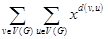(2)

In a series of papers, the Wiener index and the Hosoya polynomial of some molecular graphs and Nanotubes are computed. For more details about the Wiener index and the Hosoya polynomial, please see the paper series [4-22] and the references therein.

In this research paper, we present some properties of the Wiener index and the Hosoya polynomial and we introduce a closed formula of this and the correspondent polynomial of the Jahangir graphs J5,m for all integer number m≥3.

2. Materials and Methods

The In the this section, we present some studies about a semi-regular and connected graphs that named "Jahangir Graphs Jn,m"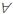n≥2,m≥3. And we introduce a method to compute the Wiener index and the Hosoya polynomial of the Jahangir graphs J5,m in continue.

What is a the Jahangir graphs Jn,m?

Definition 2.1. [23,24] The Jahangir graphs Jn,m is a graph on nm+1 vertices and m(n+1) edgesn≥2 &m≥3; i.e., a graph consisting of a cycle Cnm with one additional vertex which is adjacent to m vertices of Cnm at distance n to each other on Cnm.Figure 1. Some examples of Jahangir graphs J5,3, J5,4, J5,5, J5,6 and J5,8.

In particular,m≥3 the Jahangir graphs J5,m is a graph consisting of a cycle C5m with one additional vertex (the Center vertex c) which is adjacent to m vertices of Cnm at distance 5 to each other on C5m. Some first example of this graphs are shown in Figure 1. For more details about the Jahangir graphs Jn,m reader can see the paper series [23-33, 34-36].

3. Main Results and Discussion

In this paper, we computed a closed formula of the Wiener index and the Hosoya polynomial of the Jahangir graphs J5,m for all integer number m≥3.

Theorem 2.1. The Hosoya polynomial and the Wiener index for the Jahangir graphs J5,m for all integer number m≥3 are equal to

H(J5,m,x)=6mx1+½ (m2+13m)x2+(2m2+5m)x3+(4m2-4m)x4+(4m2-6m)x5+(2m2-5m)x6  (3)

W(J5,m)=55m2-42m.  (4)

Proof. Let J5,m be Jahangir graphsm≥3 with 5m+1 vertices and 6m edges. From Definition 2.1 and Figure 1, one can see that there are 4m vertices of the Jahangir graph J5,m with degree 2 and m vertices of J5,m with degree 3 and Center vertex c has degree m and we have three partitions of the vertex set V(J5,m) as follow

V2={vϵV(J5,m)| dv=2} → |V2|=4m   (5)

V3={vϵV(J5,m)| dv=3} → |V3|=m  (6)

Vm={cϵV(J5,m)| dc=m} → |Vm|=1   (7)

And alternatively,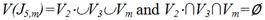and we know

|E(G)|=½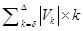(8)

where δ and Δ are the minimum and maximum of dv for all vϵV(G), respectively, thus

|E(J5,m)|=½[2×|V2 |+3×|V3|+3×|Vm|].  (9)

Now, for compute the Hosoya polynomial and the Wiener index of J5,m, we denote the number of unordered pairs of vertices u and v of a graph G as distance d(u,v)=k by d(G,k) for all integer number k up to d(G) (where d(G) denote the topological diameter and is the longest distance between vertices of a graph G). Thus, we redefine this mention topological polynomial and index of G as follow:

H(G,x)=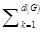d(G,k)xk  (10)

W(G)=d(G,k)×k  (11)

From the Definition 2.1 and the structure of Jahangir graphs J5,m in Figure 1, we have following computations for d(J5,m,k) (k: 1≤k≤d(J5,m)):

d(J5,m,1)=|E(J5,m)|=6m by definitions of d(G,k).  (12)

d(J5,m,2)=½m(m+13)  (13)

Since, there are two 2-edges paths between Center vertex cϵV(J5,m) and other vertices of vertex set V2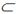V(J5,m)). ½|V3|(m-1) 2-edges paths between all vertices of u,vϵV3V(J5,m)), and 2|V3|+|V3| 2-edges paths start from vertices of V2 until vertices of V3 and V2V(J5,m). Thus the second sentence of the Hosoya polynomial H(J5,m,x) of J5,m is equal to [2|V3|+½|V3|(m-1)+3|V3|+2|V3|]x2=½(m2+13m)x2.

d(J5,m,3)=(2m2+5m)  (14)

Because, there are 2|V3| 3-edges paths between cϵVm and vertices of V2, but there are not any 3-edges paths between c and vertices of V3. Also, there are ½×2|V3|+2|V3|=3m 3-edges paths between all vertices of u,vϵV2V(J5,m)). From Figure 1, one can see that 2|V3|+|V3|(2(m-1)) 3-edges paths started from vertices of V3 until vertices of V2V(J5,m). Thus, the third sentence of H(J5,m,x) is ½ [2|V3|+0+3|V3|+2m|V3|]x3=m(2m+5)x3.

d(J5,m,4)=4m2-2m  (15)

Since from Figure 1, there are 2|V3| 3-edges paths between cϵVm and vertices of V2. And obviously there are not any 4-edges paths between vertices of V3 and c. And there are |V3|(2|V3|-4) 4-edges paths between vertices of V3 and V2. Also, there are d(Cm)+½×2|V3|(2|V3|-3) 4-edges paths between all vertices of u,vϵV2V(J5,m)). Therefore the coefficient of the fourth sentence of H(J5,m,x) is 2|V3|+0+|V3|(2|V3|-4)+2m(m-1)=4m(m-1)=4m2-4m.

d(J5,m,5)=(4m2-6m)  (16)

From the Definition 1 and the structure of J5,m, one can see that there are not any 5-edges paths between vertices of V3 and c and vertices of V2 and between vertices of uϵV2 and vϵV3. Thus for the 5th sentence of the Hosoya polynomial of J5,m, we have 2d(Cm)+½×|V2|(2|V3|-4)=2m(2m-3) 5-edges paths between all vertices of u,vϵV2.

For d(J5,m)=6; d(J5,m,6)=(2m2-5m)  (17)

Because, there isn’t any 6-edges paths started from members of C and V3 to all other vertices of Jahangir graphs J5,m in Figure 1. But there are ½×|V2|(2|V2|-5)=m(2m-5) 6-edges paths between all vertices V2. Thus, the 6th and last sentence of H(J5,m,x) is m(2m-5)x6.

Now, Equations 12, 13,…, 17 imply that the Hosoya polynomial of the Jahangir graph J5,m is equal to:

H(J5,m,x)=6mx1+½ (m2+13m)x2+(2m2+5m)x3+(4m2-4m)x4+(4m2-6m)x5+(2m2-5m)x6   (18)

Also, from the definition of Wiener index and the Hosoya Polynomial of a graph G and the first derivative of Hosoya polynomial (evaluated at x=1 in Equations 10, 11), we cam compute the Wiener index of the Jahangir graph J5,m as follow:

W(J5,m)=H(J5,m,x)’|x=1=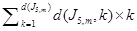=[6mx1+½ (m2+13m)x2+(2m2+5m)x3+(4m2-4m)x4+(4m2-6m)x5+(2m2-5m)x6] ’|x=1

=[6m×1+½ m(m+13)×2+m(2m+5)×3+4m(m-1)×4+m(4m-6)×5+m(2m-5)×6]=55m2-42m.   (19)

Here the proof of theorem is completed.

Example 2.1. The Hosoya polynomial and the Wiener index for J5,6 are equal to:

H(J5,6,x)=36x+57x2+102x3+120x4+108x5+42x6  (20)

W(J5,6)=55(6)2-42(6)=1728  (21)

4. Conclusion

In this paper, we obtained the Hosoya polynomial and the Wiener index of graph "the Jahangir graphs J5,m for all integer number m≥3" for the first time as:

H(J5,m,x)=6mx1+½ (m2+13m)x2+(2m2+5m)x3+(4m2-4m)x4+(4m2-6m)x5+(2m2-5m)x6

W(J5,m)=55m2-42m.

Acknowledgements

The author is thankful to Professor Emeric Deutsch from Department of Mathematics of Polytechnic University (Brooklyn, NY 11201, USA) for his precious support and suggestions.

References

1. H. Wiener, Structural determination of paraffin boiling points, J. Amer. Chem. Soc. 69 (1947), 17–20.
2. P. V. Khadikar and S. Karmarkar. On the estimation of PI index of polyacenes. Acta Chim. Slov. 49, 2002, 755-771.
3. H. Hosoya. On some counting polynomials in chemistry. Discrete Appl. Math. 19, (1988), 239-257.
4. Gutman, O. E. Polansky, Mathematical Concepts in Organic Chemistry Springer, Berlin, 1986.
5. Gutman, S. Klavžar, B. Mohar. Fifty years of the Wiener index, (Eds.), MATCH Commun. Math. Comput. Chem. 35, 1-259 (1997).
6. Gutman, S. Klavžar, B. Mohar. Fiftieth anniversary of the Wiener index, (Eds.), Discrete Appl. Math. 80, 1--113 (1997).
7. E. Estrada, O. Ivanciuc, I. Gutman, A. Gutierrez and L. Rodrguez, Extended Wiener Indices. A New Set of Descriptors for Quantitative Structure Property Studies, New J. Chem. 22 (1998) 819-823.
8. H. Hosoya, A newly proposed quantity characterizing the topological nature of structural isomers of saturated hydrocarbons, Bull. Chem. Soc. Japan 44 (1971) , 2332-2339.
9. Gutman, S. Klavžar, M. Petkovsek and P. Zigert, On Hosoya polynomials of benzenoid graphs, MATCH Commun. Math. Comput. Chem., 43 (2001) 49 - 66.
10. A. Dobrynin, R. Entringer, I. Gutman, Wiener index of trees: Theory and applications, Acta Appl. Math. 66 (2001), 211–249.
11. M. V. Diudea. Hosoya polynomial in Tori. MATCH Commun. Math. Comput. Chem. 45, (2002), 109-122.
12. M. Eliasi, B. Taeri, Hosoya polynomial of zigzag polyhex Nanotorus, J. Serb. Chem. Soc. 73 (2008) 313–319.
13. S. Xu, H. Zhang, Hosoya polynomials of armchair open–ended Nanotubes, Int. J. Quant. Chem. 107 (2007) 586–596.
14. J. Chen, S. Xu, H. Zhang, Hosoya polynomials of TUC4C8(R) Nanotubes, Int. J. Quant. Chem. 109 (2009) 641–649.
15. S. Xu, H. Zhang, The Hosoya polynomial decomposition for catacondensed benzenoid graphs, Discr. Appl. Math. 156 (2008) 2930–2938.
16. H. Shabani, A. R. Ashrafi, Applications of the matrix package MATLAB in computing the Wiener polynomial of armchair polyhex nanotubes and nanotori, J. Comput. Theor. Nanosci. 7 (2010) 1143–1146.
17. M. Knor, P. Potočnik and R. Škrekovski. Wiener index of iterated line graphs of trees homeomorphic to the claw K1;3. Ars Math. Contemp. 6 (2013), 211–219.
18. M. R. Farahani. Hosoya polynomial, Wiener and Hyper-Wiener indices of some regular graphs. Informatics Engineering, an International Journal (IEIJ), 1 (1), (2013), 9-13.
19. M. R. Farahani, M. P. Vlad. On the Schultz, Modified Schultz and the Hosoya polynomials and Derived Indices of Capra-designed planar Benzenoid. Studia UBB Chemia. 57 (4), (2012), 55-63.
20. M. R. Farahani. Schultz indices and Schultz Polynomials of Harary graph. Pacific Journal of Applied Mathematics. 6 (3), (2014), 77-84.
21. M. R. Farahani. Hosoya, Schultz, Modified Schultz Polynomials and Their Topological Indices of Benzene Molecules: First Members of Polycyclic Aromatic Hydrocarbons (PAHs). International Journal of Theoretical Chemistry. 1 (2), (2013), 09-16.
22. M. R. Farahani. On the Schultz polynomial, Modified Schultz polynomial, Hosoya polynomial and Wiener index of Circumcoronene series of benzenoid, J. Applied Math. & Info. 31 (5-6), (2013). 595-608.
23. K. Ali, E. T. Baskoro and I. Tomescu, On the Ramzey number of Pathsand Jahangir graph J3,m. 3rd International Conference on 21st Century Mathematics 2007, GC University Lahoor Pakistan, March 2007.
24. D. A. Mojdeh and A. N. Ghameshlou. Domination in Jahangir Graph J2,m.Int. J. Contemp. Math. Sciences, 2 (24), (2007), 1193-1199.
25. Lourdusamy. Covering Cover Pebbling Number for Jahangir Graph J2, m. Sciencia Acta Xaveriana. 4 (2), 2012, 59-64.
26. Lourdusamy, S. Samuel Jeyaseelan and T. Mathivanan. On Pebbling Jahangir Graph. Gen. Math. Notes, 5 (2), 2011, 42-49.
27. Lourdusamy and T. Mathivanan. The 2t-Pebbling Property on the Jahangir Graph J2 ;m. Gen. Math. Notes,28 (1), 2015, 18-39.
28. Lourdusamy and T. Mathivanan. The t-pebbling number of Jahangir graph J3,m. Proyecciones Journal of Mathematics. 34 (2), 161-174, 2015.
29. Lourdusamy, S. Samuel Jeyaseelan and T. Mathivanan. The t-Pebbling Number of Jahangir Graph. International J.Math. Combin.1, (2012), 92-95.
30. M. Ramachandran and N. Parvathi. The Medium Domination Number of a Jahangir Graph Jm,n. Indian Journal of Science and Technology, 8 (5), 400–406, 2015
31. M. R. Farahani, Hosoya Polynomial and Wiener Index of Jahangir graphs J2,m. Pacific Journal of Applied Mathematics. 7 (3), 2015, In press.
32. M. R. Farahani, The Wiener Index and Hosoya polynomial of a class of Jahangir graphs J3,m. Fundamental Journal of Mathematics and Mathematical Science. 3 (1), 2015, 91-96.
33. M. R. Farahani, Hosoya Polynomial of Jahangir graphs J4,m. Global Journal of Mathematics, 3 (1), 2015, 232-236.
34. S. Wang, B. Wei, Multiplicative Zagreb indices of Cactus graphs, Discrete Mathematics, Algorithms and Applications, DOI: 10.1142/S1793830916500403.
35. C. Wang, S. Wang, B. Wei, Cacti with Extremal PI Index, Transactions on Combinatorics, Vol. 5 No. 4 (2016), pp. 1-8.
36. S. Wang, B. Wei, Multiplicative Zagreb indices of k-trees, Discrete Applied Mathematics, 180 (2015), 168-175.

 Contents 1. 2. 3. 4.
Article ToolsAbstractPDF(424K)